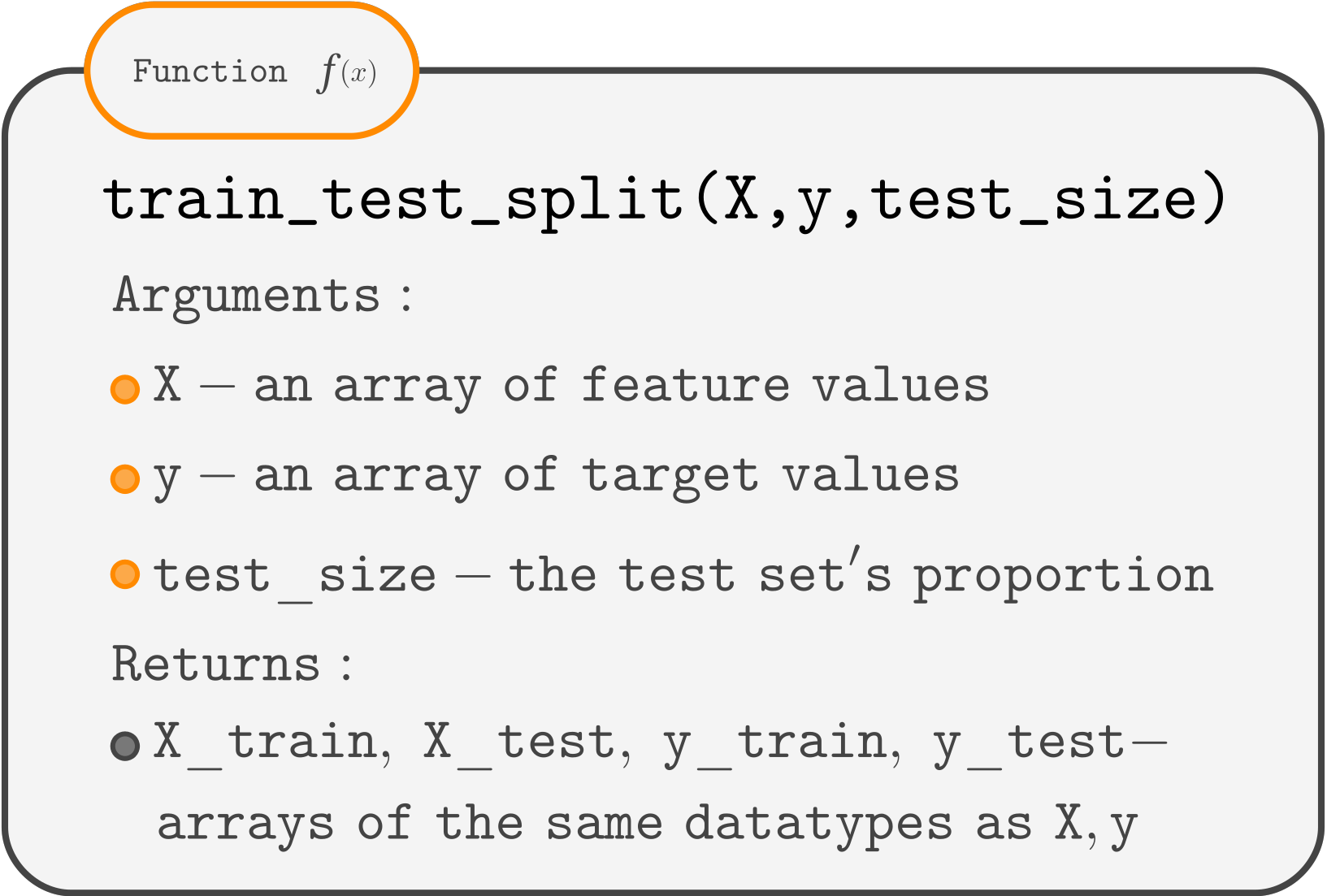Course Content

ML Introduction with scikit-learn

##Evaluating a Model. Train-Test split.

When we build a model for predictions, it is essential to understand how well the model performs before actually predicting something.
Evaluating a model refers to a process of assessing how well it performs in making predictions.
That's why the `.score()` method is needed.
But if we evaluate the model using the training set data, the results are unreliable since a model is likely to perform better on the data it was trained on rather than on the data it has never seen.
So it is crucial to evaluate the model on the data it has never seen to understand how well it will perform.

We can do this by randomly splitting the data into a training set and a test set.

Now we can train a model on a training set and evaluate its performance on a test set.

To randomly split the data, we can use the `train_test_split()` function from the `sklearn.model_selection` module.Usually, for a test set, we use 25-40% of data when the dataset is small, 10-30% for a medium dataset, and <10% for large datasets.
In our example, there are only 342 instances, a small dataset. So we will use 33% as a test set.
Here is a syntax:

We call a training set `X_train, y_train` and a test – `X_test, y_test`.Notice that now we use the training set in the `.fit(X_train, y_train)` and the test set in the `.score(X_test, y_test)`.
Since the `train_test_split()` splits the dataset randomly, each time you press a Run Code button, there are different train and test sets. You can press it several times and see that the scores differ. These scores would become more stable if the dataset's size increased.